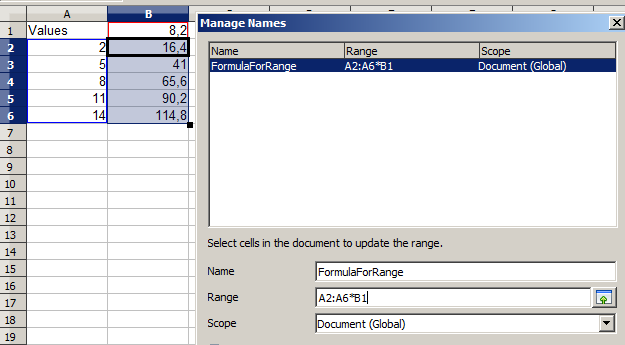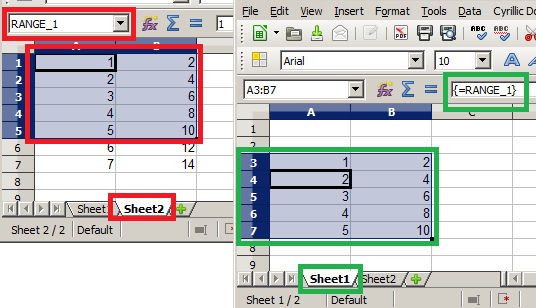# Calc: Copy and paste named ranges

Is there a way to copy and paste the cell contents of a named range in another worksheet, by a given cell value. for exaple

Firts of all “RANGE_1” is at sheet 2 (A1:B5) A column is text B column are numbers

In sheet 1 cell a1 contains the text “RANGE_1”
I whant to have a macro triggered by a button that when pressed it gets the range name from cell a1 at sheet 1 then it goes to sheet 2 gets the contents from the existing RANGE_1

an paste all in sheet 1 starting at A3

Is this even possible?

Thank you JohnSUN, it works just like you said, thank you very much. I guess I asked the wrong question. Let see if I explain my self better this time. Range_1 is in sheet 2 - A2:B6, and the B column has some formulas that use sheet 2 - cell B1(the one directly above the second column of the range), what I want to accomplish is to have Range_1 pasted at sheet 1 cell A3 and have the the text in the A column and the B column formulas in the range interact with the cell just above the newly pasted range, so if I paste Range_1 in C45 the formulas will interact with with D44. Like doing a manual copy of A2:B6 and pasting special >> text, formulas and numbers

I guess a file is worth 100words, in sheet one 1(MAIN) I want to click on Cell B5 to set it as current, then select a name from the combo box, then click on the push button to run a macro to copy&paste at active cell the named range in the combo box. examp >https://www.box.com/s/ayv08oftb9e17lg6yy6j

I think I have to copy the range values and formulas and then paste but I can figure out how also I found this example but I just cant implemented>> http://www.oooforum.org/forum/viewtopic.phtml?t=59919

Of course it is possible. But it requires a good understanding of formulas Calc. You did not say what formula would be used. Suppose you want to get a product of every left cell with the value of the top cell. That is for your example it would be formulas:

``````#    A       B
1 Values    8,2
2    2    =B1*A2=16,4
3    5    =B1*A3=41
4    8    =B1*A4=65,6
5   11    =B1*A5=90,2
6   14    =B1*A6=114,8
``````

Now just select range B2:B6 and press Ctrl+F3.

Attention! Active cell (with black border) must be B2!Add new named range with name, for example, “FormulaForRange”. At field Range write formula

``````A2:A6*B1
``````

Now fill range E24:E28 (or J6:J10, or AKG533:AKG537…) with some values, set coefficient at F23(or K5, or AKH532…). In cell F24 (or K6, or AKH533…) type
=FormulaForRange and press Ctrl+Shift+Enter

It is not difficult. But copy a range of cells, and Ctrl+Shift+V->Formulas easier

Yes, of course it possible

When describing RANGE_1 use absolute addresses (with dollar signs) - \$Sheet2.\$A\$1:\$B\$5

Select cell A3 on Sheet1, type

``````=RANGE_1
``````

and press Ctrl+Shift+Enter

The formula in cell A3:B7 is {=RANGE_1} and show values from named range RANGE_1Excellent explanation!
Why is crtl+shift+enter needed?

Because this is an array formula

Thanks, I learned something. Haven’t used yet any array formula.